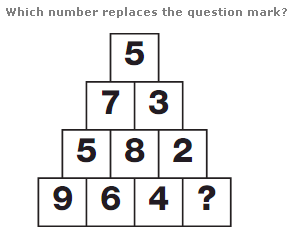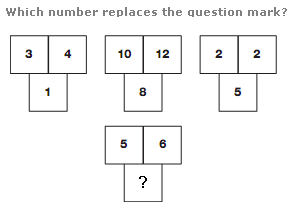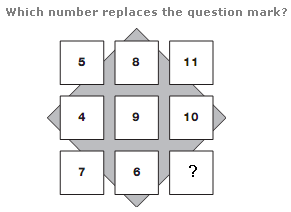# Puzzles - Number puzzles

### Exercise :: Number puzzlesAnswer : 1 Explanation : Starting with the bottom line of the triangle and moving up, the sum of the digits in each line decreases by 5 each time, from 20 to 5.Answer : 2 Explanation : Numbers in the squares of the top middle figure equal the sum of the numbers in corresponding squares of the other 3 figures.Answer : 13 Explanation : Starting at the top left and working down, then to the right and up, and finally to the right and down in a zig-zag shape, numbers follow the repeated sequence, subtracting 1 then adding 3 etc.